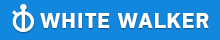GMAT Question of the Day - Daily to your Mailbox; hard ones only

 It is currently 26 Jun 2019, 11:22GMAT Club Daily Prep

Thank you for using the timer - this advanced tool can estimate your performance and suggest more practice questions. We have subscribed you to Daily Prep Questions via email.

Customized
for You

we will pick new questions that match your level based on your Timer History

Track

every week, we’ll send you an estimated GMAT score based on your performance

Practice
Pays

we will pick new questions that match your level based on your Timer History

Not interested in getting valuable practice questions and articles delivered to your email? No problem, unsubscribe here.Which of the following is NOT equal to 0.0675?

Author Message
TAGS:

Hide Tags

Math ExpertV
Joined: 02 Sep 2009
Posts: 55803
Which of the following is NOT equal to 0.0675?  [#permalink]

Show Tags00:00

Difficulty:5% (low)

Question Stats:86% (00:49) correct14% (00:58) wrongbased on 40 sessions

HideShow timer StatisticsWhich of the following is NOT equal to 0.0675?

A. $$67.5*10^{−3}$$

B. $$6.75*10^{−2}$$

C. $$0.675 * 10^{−1}$$

D. $$0.00675 * 10^2$$

E. $$0.0000675 * 10^3$$

_________________
VPD
Status: Learning stage
Joined: 01 Oct 2017
Posts: 1010
WE: Supply Chain Management (Energy and Utilities)
Re: Which of the following is NOT equal to 0.0675?  [#permalink]

Show Tags

Bunuel wrote:
Which of the following is NOT equal to 0.0675?

A. $$67.5*10^{−3}$$

B. $$6.75*10^{−2}$$

C. $$0.675 * 10^{−1}$$

D. $$0.00675 * 10^2$$

E. $$0.0000675 * 10^3$$

Let's travel thru options:-

A. $$67.5*10^{−3}$$ ; shift the decimal by 3 positions to the left, we have 0.0675

B. $$6.75*10^{−2}$$ ; shift the decimal by 2 positions to the left, we have 0.0675

C. $$0.675 * 10^{−1}$$ ; shift the decimal by 1 positions to the left, we have 0.0675

D. $$0.00675 * 10^2$$ ; shift the decimal by 2 positions to the right, we have 0.675

E. $$0.0000675 * 10^3$$; shift the decimal by 3 positions to the right, we have 0.0675

Ans. (D)
_________________
Regards,

PKN

Rise above the storm, you will find the sunshineRe: Which of the following is NOT equal to 0.0675?   [#permalink] 25 Dec 2018, 01:12
Display posts from previous: Sort by

Which of the following is NOT equal to 0.0675?